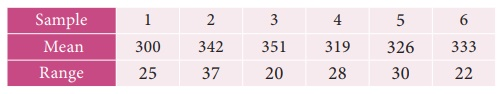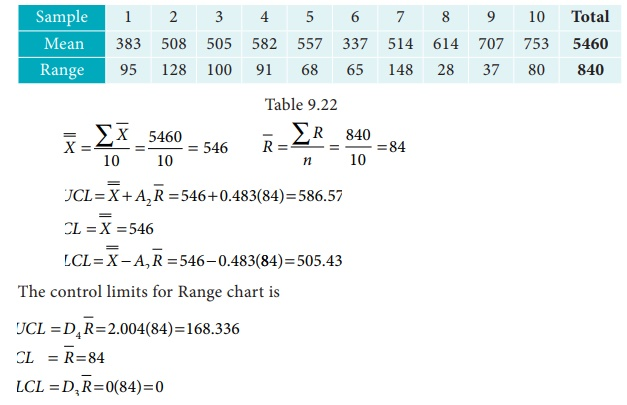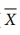Home | | Business Maths 12th Std | Statistical Quality Control (SQC): Example Solved Problems

# Statistical Quality Control (SQC): Example Solved Problems

Applied Statistics: Statistical Quality Control (SQC)

Example 9.19

A machine drills hole in a pipe with a mean diameter of 0.532 cm and a standard deviation of 0.002 cm. Calculate the control limits for mean of samples 5.

Solution:Example 9.20

The following data gives the readings for 8 samples of size 6 each in the production of a certain product. Find the control limits using mean chart.Given for n = 6, A2 = 0.483,

Solution:Example 9.21

The data shows the sample mean and range for 10 samples for size 5 each. Find the control limits for mean chart and range chart.Solution:Example 9.22

The following data gives readings of 10 samples of size 6 each in the production of a certain product. Draw control chart for mean and range with its control limits.Solution:Example 9.23

You are given below the values of sample mean () and the range ( R ) for ten samples of size 5 each. Draw mean chart and comment on the state of control of the process.Given the following control chart constraint for : n = 5, A2 = 0.58, D3 = 0 and D4 = 2.115

Solution:The above diagram shows all the three control lines with the data points plotted, since four points falls out of the control limits, we can say that the process is out of control.

Tags : with Answer, Solution | Applied Statistics , 12th Business Maths and Statistics : Chapter 9 : Applied Statistics
Study Material, Lecturing Notes, Assignment, Reference, Wiki description explanation, brief detail
12th Business Maths and Statistics : Chapter 9 : Applied Statistics : Statistical Quality Control (SQC): Example Solved Problems | with Answer, Solution | Applied Statistics